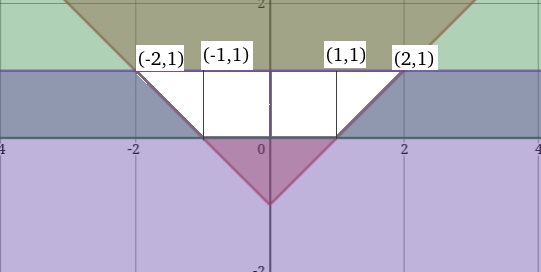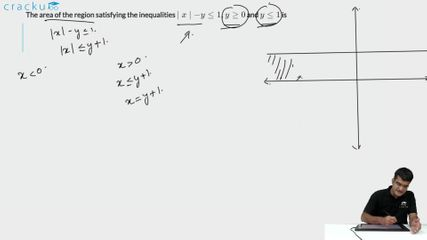Question 7

# The area of the region satisfying the inequalities $$\mid x\mid-y\leq1,y\geq0$$ and $$y\leq1$$ is

SolutionThe area of the region contained by the lines $$\mid x\mid-y\leq1,y\geq0$$ and $$y\leq1$$ is the white region.

Total area = Area of rectangle + 2 * Area of triangle = $$2+\left(\frac{1}{2}\times\ 2\times\ 1\right)\ =3$$

Hence, 3 is the correct answer.

### View Video Solution